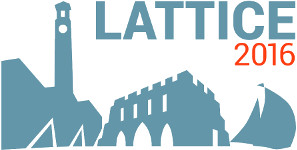#The 34th International Symposium on Lattice Field Theory (Lattice 2016)

Jul 24 – 30, 2016
Highfield Campus, University of Southampton
Europe/London timezone

## A study of the radiative transition $\pi \pi \to \pi \gamma^{\ast}$ with lattice QCD

Jul 29, 2016, 5:50 PM
20m
Building B2a Room 2077 (Highfield Campus, University of Southampton)

### Speaker

Dr Luka Leskovec (University of Arizona)

### Description

Lattice QCD calculations of radiative transitions between hadrons have in the past been limited to processes of hadrons stable under the strong interaction. Recently developed methods for $1 \to 2$ transition matrix elements in a finite volume now enable the determination of radiative decay rates of strongly unstable particles. Our lattice QCD study focuses on the process $\pi \pi \to \pi \gamma^{\ast}$, where the $\rho$ meson is present as an enhancement in the cross-section. We use 2+1 flavors of clover fermions, initially at a pion mass of approximately 320 MeV and a lattice size of approximately 3.6 fm. The required 2-point and 3-point correlation functions are constructed from a set of forward, sequential and stochastic light quark propagators. In addition to determining the $\rho$ meson resonance parameters via the Lüscher method, the scattering phase shift is used in concert with the $1 \to 2$ transition matrix element formalism of Briceño et al. to compute the $\pi \pi \to \pi \gamma^{\ast}$ amplitude at several values of the momentum transfer and $\pi\pi$ invariant mass.

### Primary authors

Prof. Andrew Pochinsky (MIT) Prof. Constantia Alexandrou (University of Cyprus and The Cyprus Institute) Dr Giannis Koutsou (The Cyprus Institute) Mr Gumaro Rendon (University of Arizona) Prof. John Negele (MIT) Dr Luka Leskovec (University of Arizona) Dr Marcus Petschlies (University of Bonn) Mr SRIJIT PAUL (HPC-LEAP) Prof. Sergey Syritsyn (Jefferson Lab) Prof. Stefan Meinel (RIKEN BNL, University of Arizona)

 Slides